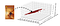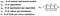# How to Evaluate Text Readability with NLP

Reader engagement is a recurrent problem among all types of readers: adults/children and teachers/students. They all face the same issue: finding books close to their current readability ability, either for casual reading (easy level) or to improve and learn (hard level) without being flooded by too much difficulty which usually results in a harsh experience for most of us.

At Glose, our goal is to enable readers to access books that are gradually more difficult and recommend books that fit their current reading ability.

In this article, we will show how we developed a machine learning system that objectively evaluates text readability.

# Text Complexity: Facets and Usage

Text complexity measurement estimates how difficult it is to understand a document. It can be defined by two concepts: legibility and readability.

Legibility ranges from character perception to all the nuances of formatting such as bold, font style, font size, italic, word spacing …

Readability, on the other hand, focuses on textual content such as lexical, semantical, syntactical and discourse cohesion analysis. It is usually computed in a very approximate manner, using average sentence length (in characters or words) and average word length (in characters or syllables) in sentences.

A few other text complexity features do not depend on the text itself, but rather on the reader’s intent (homework, learning, leisure, …) and cognitive focus (which can be influenced by ambient noise, stress level, or any other type of distraction).

Why is it crucial to be able to measure text readability ?

In the context of conveying important information to most readers (drugs leaflets, news, administrative and legal documents), an evaluation of readability helps text writers to adjust their content to their target audience’s level.

Another use case is the field of automatic text simplification, where a robust readability metric can replace standard objective functions (such as a mixture of BLEU and Flesch-Kincaid) used to train text simplification systems.

In this article, we will focus solely on estimating text readability using annotated datasets and machine learning algorithms. We implemented them using the scikit-learn framework.

# Data

The starting point of any machine learning task is to collect data. In our case, we extract it from two sources:

1. Our database at Glose contains more than 1 million books which include around 800,000 english books.
2. A dataset of 330,000 book identifiers, graded on the Lexile text complexity scale ∈ [-200, 2200].

This dataset is biased in two ways:

1. The distribution of book genres in our merged dataset is unbalanced (figure above).
2. It assumes that the Lexile score is close to the true readability perception of the average human, which might not be, due to their usage of mainly two features: sentence length and words frequency.

Book identifiers (namely ISBN), are unique to a book’s edition. Each book can have multiple ISBNs due to the large number of editors distributing the same content. In short, each identifier in our dataset maps to multiple identifiers of similar content.

In order to have a unique mapping between ISBN, book content, and Lexile score, we select an intersection subset (where we have both a book’s content in our database and a Lexile annotation) of 17,000 english books.

# Book representation

In the first step of our natural language processing pipeline, we clean and tokenize the text into sentences and words. Then we have to represent text as an array of numbers (a.k.a. feature vector): here we choose to represent text by hand-crafted variables in order to embed higher level meaning than a sequence of raw characters.

Each book is represented by a vector of 50 float numbers, each of them being a text feature such as:Spacial representation of 3 features (out of 50) for 1000 data points. The Dune book position is indicated by the arrow.

These features are all on different scales (c.f. figure above), however we would like to have a similar scale from -1 to 1 because some of the algorithms we use during modelling (Support Vector Regression with a Linear kernel and Linear regression) assume that the data given as input follows a Gaussian distribution. This process, namely standardisation, is about removing the mean and dividing by the standard deviation of a dataset.

# Feature selection

Now that we built a set of features representing a text, we would like to truncate that vector to the most salient features ; the ones that discriminate the most our annotations. Using features that do not carry information related to the target variable (the readability score) is a computation time burden to the model, because the inference is done with more features than necessary.

To perform this feature selection step, we use the LASSO method (scikit-learn implementation) with cross-validation (CV is the process of training and testing models with different data splits to avoid a bias from a specific dataset order) because the difference between execution time with and without 10-fold CV is negligible. Moreover, it guarantees to have a model that is less subject to variance when confronted to real data.

The LASSO method is performed by creating multiple subsets of our feature set. For each feature set a regression function is fitted using our training data. Then a correlation is computed (using a metric such as Person, Kendall-Tau or Chi-Square) between each set’s regression function and the readability score. Feature sets are ranked by correlation performance and the best one is selected.

# Choosing the right model

Our output variable is numerical and continuous which narrows the spectrum of machine learning models applicable to our dataset (regression task). To select an appropriate model, there is several indicators that may guide one’s choice, such as the number of features or the number of samples available.

In the case of constrained bayesian algorithms such as Naive Bayes variants (simple or tree augmented), performances are likely to decrease with large number of features. This is due to their inability to build large variable dependencies between an output variable and an explanatory variable. Naive Bayes is built under the assumption that variables are independent, which is less likely the case with longer feature vectors. Tree Augmented Naive Bayes (TAN) allows only one explanatory variable as a dependency of another to predict an output variable. This lack of feature intrication makes these algorithms bad candidates for our feature vector length (50), we will not use them in this article.

However, Decision Tree (DT) based algorithms cope very well with high dimensional data (more features) but need lots of data samples (varies as a function of algorithm hyperparameters). DTs build rules (for example: average number of words per sentence > 5) and these rules are split when a given amount of data samples fit them. For example: 10 samples fit the previous rule, we consider that there is too much samples in this rule, so we build two other rules > 5 AND < 10 and > 10 where we fit respectively 4 and 6 samples instead of 10 in one rule. In decision tree algorithms, the number of data samples is a function of model granularity, by handling overfitting correctly, the more data and features there is, the better a DT based model is.

Another approach to model selection that we choose to use is Grid Search, this technique is a training and testing brute force over a set of models and a set of hyper parameters for each model.

Pros: Easy to setup, less preliminary analysis of dataset, specific model knowledge isn’t much needed, empirical evidence (you won’t know unless you try).

Cons: Hyper parameter sets definition needs specific model knowledge and literature review to reduce computation time, time-consuming search (e.g. next figure), no global optimum guarantee.Number of complete training and testing iterations during grid search CV

In our Grid Search, three algorithms compete: a Random Forest Regressor (4 hyper parameters), a Linear Regression and a Support Vector Regressor (2 hyper parameters), the best model is generated through Random Forest regression.

We now have a production grade model that takes a book’s feature vector (obtained through pre-processing) as input and gives a readability score as output. In order to display a comprehensible metric to users (especially pre-college students), we would like to have a more meaningful representation of this score by converting it to grade level bins, we use the following formula to define those bins.

On the following figure we can see the most interesting sections of the readability scale for the students that will read their books on Glose. A teacher can follow a student’s progression on this scale by monitoring the mean grade level of the books he reads.

# Performance

Overall our best model achieves around 0.88 for the metric R² which explains 88% of our test set variance. R², also known as coefficient of determination, is the metric we use to test our regression algorithm. The resulting value we get from it ranges from 0 to 1 and Random Forest is optimised to converge to 1. This value is the explained variance accounted by our model: the higher it is, the less test data samples we find outside of our model’s prediction error range.

On the figure above we see that most of our predictions (60%) fall in the right grade level, whereas nearly 35% in only one grade level above or below ground truth. Adjacent precision is equal to 95%, this metric is more relaxed than precision as it allows up to one grade level error.

However, when we inspect the residuals per grade level and the distribution of grade levels over our test set, we realise that most of our errors (yellow, orange and red bars) happen on grade levels with fewer samples (levels 7 to 12 included).

Statistically, our results seem satisfactory. However we have room for improvement with this approach and we are going to evaluate the robustness of our model with human experts giving their feedback in the loop.

# Conclusion and outlook

As a TL;DR and a takeaway of this post, you should have learned:

As a premise of our next article, we are currently working on another approach to evaluate text readability using neural language models as comprehension systems to infill Cloze tests (text chunks with blank words). The training phase of this other approach is unsupervised and has the advantage of being language agnostic.

Written by

Written by

## Marc Benzahra

#### NLP Engineer & PhD candidate @Glose & @LIMSI_NLP